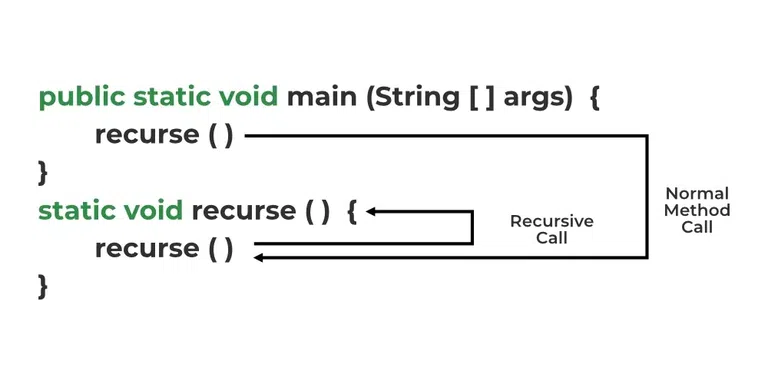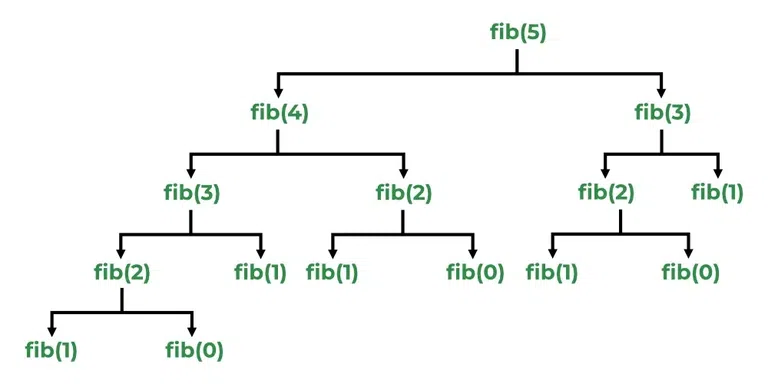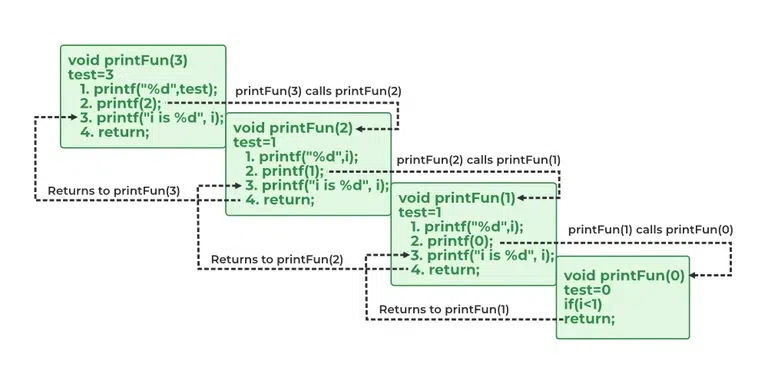Open In App
Related Articles

# Recursion in Java

In Java, Recursion is a process in which a function calls itself directly or indirectly is called recursion and the corresponding function is called a recursive function. Using a recursive algorithm, certain problems can be solved quite easily. A few Java recursion examples are Towers of Hanoi (TOH), Inorder/Preorder/Postorder Tree Traversals, DFS of Graph, etc.

### Base Condition in Recursion

In the recursive program, the solution to the base case is provided and the solution to the bigger problem is expressed in terms of smaller problems.

```int fact(int n)
{
if (n < = 1) // base case
return 1;
else
return n*fact(n-1);
}```

In the above example, the base case for n < = 1 is defined and the larger value of a number can be solved by converting it to a smaller one till the base case is reached.

### Working of Recursion

The idea is to represent a problem in terms of one or more smaller sub-problems and add base conditions that stop the recursion. For example, we compute factorial n if we know the factorial of (n-1). The base case for factorial would be n = 0. We return 1 when n = 0.## Java Recursion Programs

### 1. Factorial Using Recursion

The classic example of recursion is the computation of the factorial of a number. The factorial of a number N is the product of all the numbers between 1 and N. The below-given code computes the factorial of the numbers: 3, 4,  and 5.

• 3=  3 *2*1 (6)
• 4=  4*3*2*1 (24)
• 5=  5*3*2*1 (120)

Below is the implementation of the factorial:

## Java

 `// Java Program to implement``// Factorial using recursion``class` `GFG {` `    ``// recursive method``    ``int` `fact(``int` `n)``    ``{``        ``int` `result;` `        ``if` `(n == ``1``)``            ``return` `1``;``        ``result = fact(n - ``1``) * n;``        ``return` `result;``    ``}``}` `// Driver Class``class` `Recursion {` `    ``// Main function``    ``public` `static` `void` `main(String[] args)``    ``{``        ``GFG f = ``new` `GFG();` `        ``System.out.println(``"Factorial of 3 is "``                           ``+ f.fact(``3``));``        ``System.out.println(``"Factorial of 4 is "``                           ``+ f.fact(``4``));``        ``System.out.println(``"Factorial of 5 is "``                           ``+ f.fact(``5``));``    ``}``}`

Output

```Factorial of 3 is 6
Factorial of 4 is 24
Factorial of 5 is 120```

### 2. Fibonacci Series

Fibonacci Numbers are the numbers is the integer sequence where Fib(N) = Fib(N-2) + Fib(N-1). Below is the example to find 3,4,5.

• Fib(3) = Fib(2) + Fib(1) = Fib(1) + 0 + 1 = 1+1 = 2
• Fib(4) = Fib(3) + Fib(2) = 2+1 = 3
• Fib(5) = Fib(4) + Fib(3) = 3 + 2 = 5Recursion Tree Diagram for Fibonacci Series

Below is the implementation of the Fibonacci Series:

## Java

 `// Java Program to implement``// Fibonacci Series``import` `java.io.*;` `// Driver Function``class` `GFG {` `    ``// Function to return Fibonacci value``    ``static` `int` `Fib(``int` `N)``    ``{``        ``if` `(N == ``0` `|| N == ``1``)``            ``return` `N;` `        ``return` `Fib(N - ``1``) + Fib(N - ``2``);``    ``}` `    ``// Main function``    ``public` `static` `void` `main(String[] args)``    ``{``        ``// Factorial of 3``        ``System.out.println(``"Factorial of "` `+ ``3` `+ ``" "``                           ``+ Fib(``3``));` `        ``// Factorial of 4``        ``System.out.println(``"Factorial of "` `+ ``4` `+ ``" "``                           ``+ Fib(``4``));` `        ``// Factorial of 3``        ``System.out.println(``"Factorial of "` `+ ``5` `+ ``" "``                           ``+ Fib(``5``));``    ``}``}`

Output

```Factorial of 3 2
Factorial of 4 3
Factorial of 5 5```

## Stack Overflow error

If the base case is not reached or not defined, then the stack overflow problem may arise. Let us take an example to understand this.

```int fact(int n)
{
// wrong base case (it may cause
// stack overflow).
if (n == 100)
return 1;
else
return n*fact(n-1);
}```

If fact(10) is called, it will call fact(9), fact(8), fact(7) and so on but the number will never reach 100. So, the base case is not reached. If the memory is exhausted by these functions on the stack, it will cause a stack overflow error.

## How is memory allocated to different function calls in recursion?

When any function is called from main(), the memory is allocated to it on the stack. A recursive function calls itself, the memory for the called function is allocated on top of memory allocated to the calling function and a different copy of local variables is created for each function call. When the base case is reached, the function returns its value to the function by whom it is called and memory is de-allocated and the process continues.

Let us take the example of recursion by taking a simple function.

## Java

 `// A Java program to demonstrate``// working of recursion` `class` `GFG {``    ``static` `void` `printFun(``int` `test)``    ``{``        ``if` `(test < ``1``)``            ``return``;` `        ``else` `{``            ``System.out.printf(``"%d "``, test);` `            ``// Statement 2``            ``printFun(test - ``1``);` `            ``System.out.printf(``"%d "``, test);``            ``return``;``        ``}``    ``}` `    ``public` `static` `void` `main(String[] args)``    ``{``        ``int` `test = ``3``;``        ``printFun(test);``    ``}``}`

Output

`3 2 1 1 2 3 `

### Explanation of the above Program

When printFun(3) is called from main(), memory is allocated to printFun(3), a local variable test is initialized to 3, and statements 1 to 4 are pushed on the stack as shown below diagram. It first prints ‘3’.

In statement 2, printFun(2) is called and memory is allocated to printFun(2), a local variable test is initialized to 2, and statements 1 to 4 are pushed in the stack. Similarly, printFun(2) calls printFun(1) and printFun(1) calls printFun(0). printFun(0) goes to if statement and it return to printFun(1).

The remaining statements of printFun(1) are executed and it returns to printFun(2) and so on. In the output, values from 3 to 1 are printed and then 1 to 3 are printed.

The memory stack is shown in the below diagram:Memory Stack Representation of Java Recursive Program

The advantages of recursive programs are as follows:

• Recursion provides a clean and simple way to write code.
• Some problems are inherently recursive like tree traversals, Tower of Hanoi, etc. For such problems, it is preferred to write recursive code.

The disadvantages of recursive programs is as follows:

• The recursive program has greater space requirements than the iterative program as all functions will remain in the stack until the base case is reached.
• It also has greater time requirements because of function calls and returns overhead.

Note: Both recursive and iterative programs have the same problem-solving powers, i.e., every recursive program can be written iteratively and vice versa is also true.

## FAQs on Java Recursion

### Q1. What is recursion in Java?

Ans:

Recursion is the process where a function self-calls itself to find the result. The base condition acts as the breakpoint to the self-calling process.

### Q2. What is the difference between direct and indirect recursion?

Ans:

A function fun is called direct recursive if it calls the same function fun. A function fun is called indirect recursive if it calls another function say fun_new and fun_new calls fun directly or indirectly. The difference between direct and indirect recursion has been illustrated in Table 1.

i) . Direct recursion:

```void directRecFun()
{
// Some code....
directRecFun();
// Some code...
}```

ii) . Indirect recursion:

```void indirectRecFun1()
{
// Some code...
indirectRecFun2();
// Some code...
}

void indirectRecFun2()
{
// Some code...
indirectRecFun1();
// Some code...
}```

### Q3. What is the difference between tailed and non-tailed recursion?

Ans:

A recursive function is a tail recursive when a recursive call is the last thing executed by the function. Please refer tail recursion article for details.